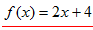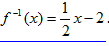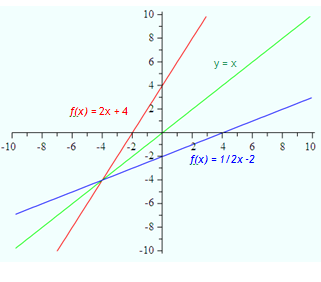Finding an Inverse

An inverse function is a function that undoes another function, much like addition undoes subtraction. Inverse functions use inverse operations to turn inputs into outputs and vice versa.

The function, f -1 (x), is defined as the inverse function of f(x) if it consistently reverses the process of f(x). That is, if f(x) turns a into b, then f -1(x) must turn b into a. f -1 (x) is not an exponent and is read "the inverse of function f." It is meant to be an inverse function, not a reciprocal.

There are a couple of ways to think about the inverse of a function. Inverses can be approached by looking at graphs or performing algebraic operations. In either case, it comes down to the basic notion that the inverse of a function reverses the x and y coordinates. In other words, for every ordered pair (x, y) in a function there will be an ordered pair (y, x) in the inverse function.

The graph of an inverse of a function is reflected over the line y = x. Reflecting over the line y = x produces the goal of reversing the x and y coordinates.

In the graph below, the original functionis reflected over the line y =x and gives us the inverse function.